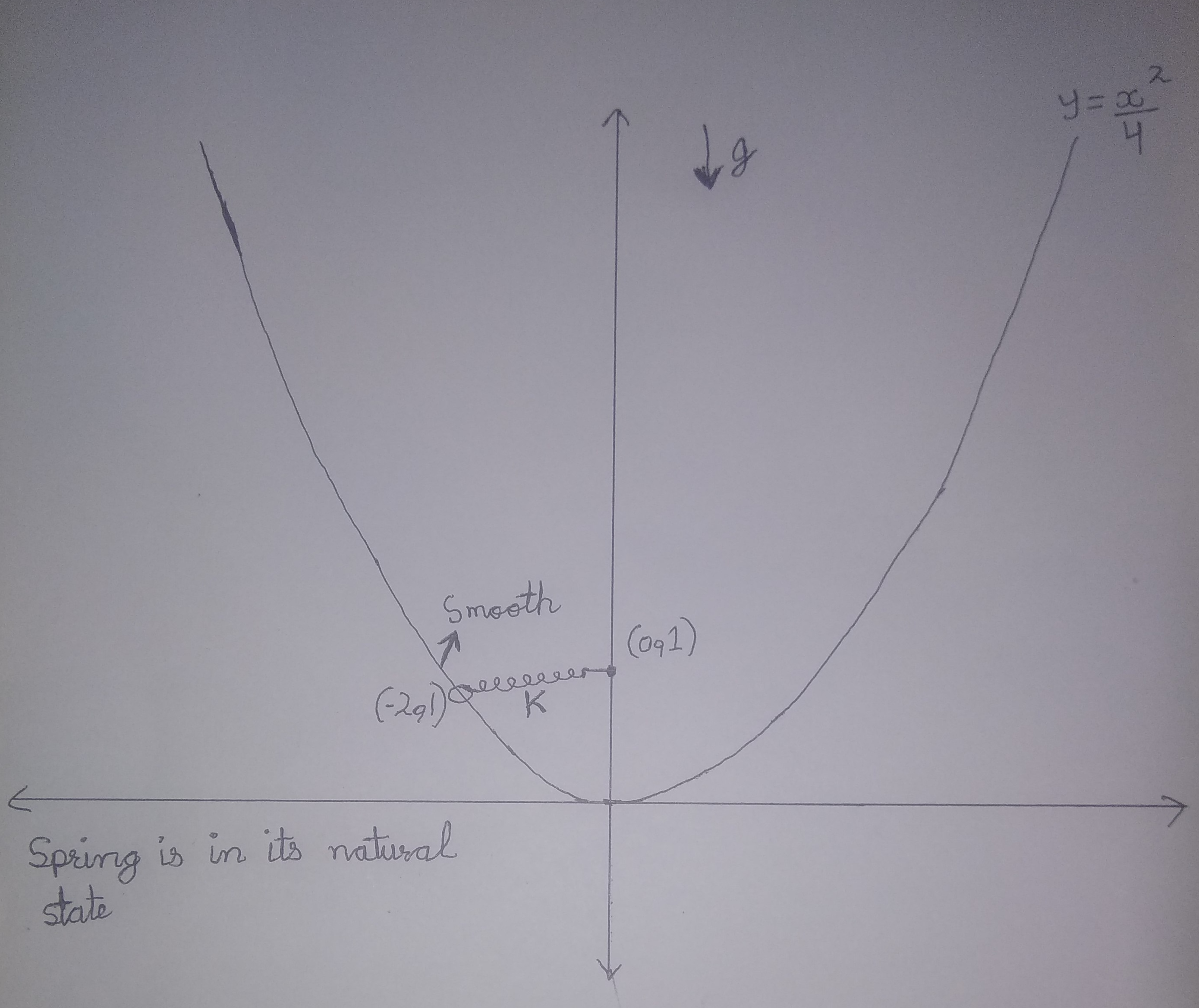# Graphical Mechanics (Part 3)A ring of mass $m$ is connected with a spring of stiffness $k$ whose other end is $\text{fixed}$ as shown. Ring is constrained to move along $\text{wedge}$ shaped $\displaystyle y = \frac{x^2}{4}$. The system is released from $\text{rest}$. Find its Time Period $t$ in $\text{seconds}$.

If $\displaystyle t = \sqrt{\frac{a}{b}} × \pi \text{ where } a, b \in \N \text{ and } \gcd(a, b) = 1$, enter answer as $a + b$.

# Details and Assumptions

• All surfaces are $\color{#3D99F6}{\text{smooth}}$

• Take acceleration due to gravity $\color{#3D99F6}{g = 10m/s^2}$

• Spring is $\color{#3D99F6}{\text{ideal}}$ and is initially in its $\color{#3D99F6}{\text{natural state}}$

• Stiffness of spring $\color{#3D99F6}{k = mg \text{ N/m}}$

• Initially, ring is at $\color{#3D99F6}{(-2, 1)}$ and other end of spring is fixed at $\color{#3D99F6}{(0, 1)}$

Inspiration Aniket Sanghi

Try similar problems by me

All of my problems are original

×